# CIS 2033 A Modern Introduction to Probability and

• Slides: 10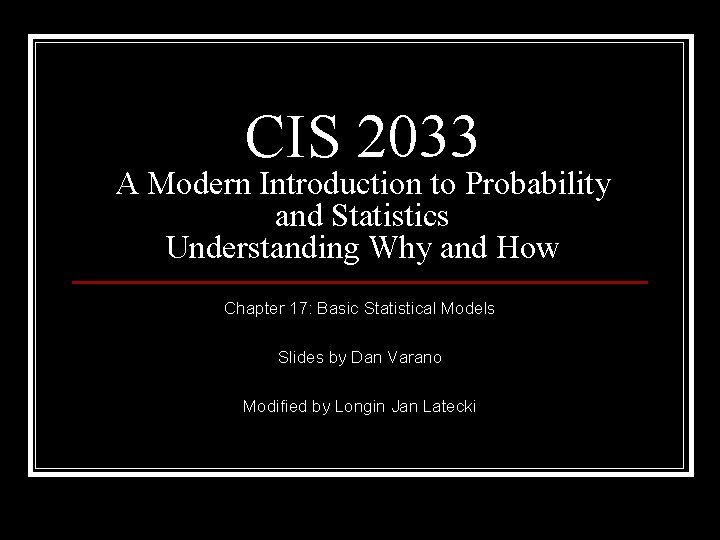CIS 2033 A Modern Introduction to Probability and Statistics Understanding Why and How Chapter 17: Basic Statistical Models Slides by Dan Varano Modified by Longin Jan Latecki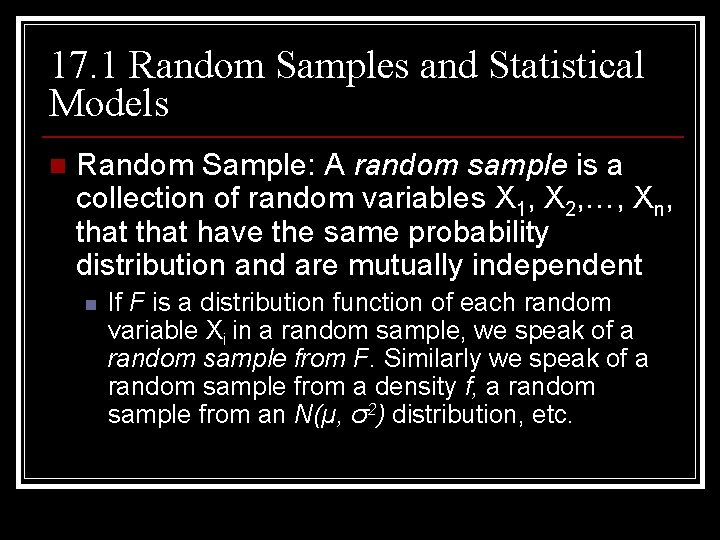17. 1 Random Samples and Statistical Models n Random Sample: A random sample is a collection of random variables X 1, X 2, …, Xn, that have the same probability distribution and are mutually independent n If F is a distribution function of each random variable Xi in a random sample, we speak of a random sample from F. Similarly we speak of a random sample from a density f, a random sample from an N(µ, σ2) distribution, etc.17. 1 continued n Statistical Model for repeated measurements n A dataset consisting of values x 1, x 2, …, xn of repeated measurements of the same quantity is modeled as the realization of a random sample X 1, X 2, …, Xn. The model may include a partial specification of the probability distribution of each X i.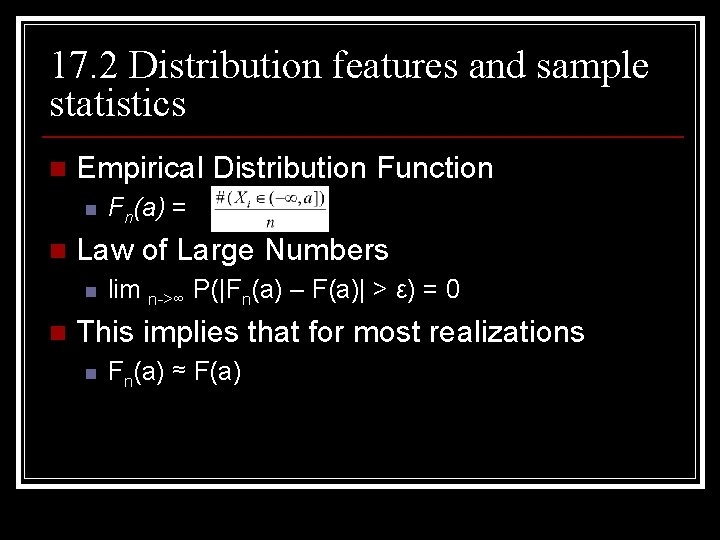17. 2 Distribution features and sample statistics n Empirical Distribution Function n n Law of Large Numbers n n Fn(a) = lim n->∞ P(|Fn(a) – F(a)| > ε) = 0 This implies that for most realizations n Fn(a) ≈ F(a)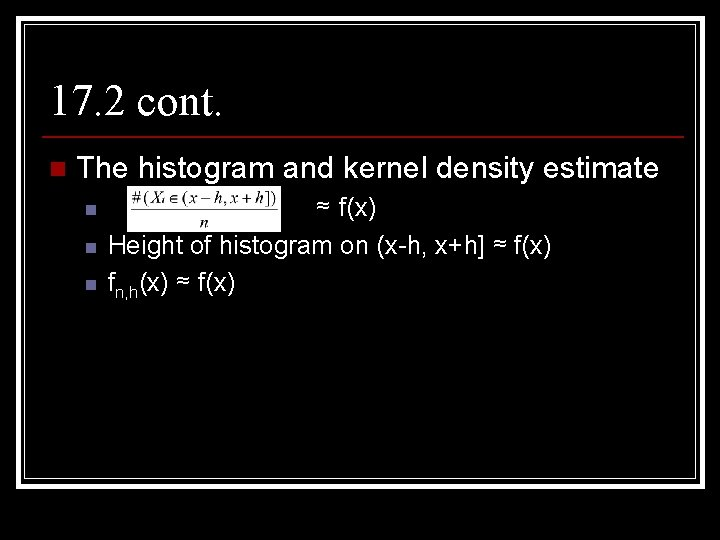17. 2 cont. n The histogram and kernel density estimate n n n ≈ f(x) Height of histogram on (x-h, x+h] ≈ f(x) fn, h(x) ≈ f(x)17. 2 cont. n The sample mean, sample median, and empirical quantiles n n n Ẋn ≈ µ Med(x 1, x 2, …, xn) ≈ q 0. 5 = Finv(0. 5) qn(p) ≈ Finv(p) = qp17. 2 cont. n The sample variance and standard deviation, and the MAD n n Sn 2 ≈ σ2 and Sn ≈ σ MAD(X 1, X 2, …, Xn) ≈ Finv(0. 75) – Finv (0. 5)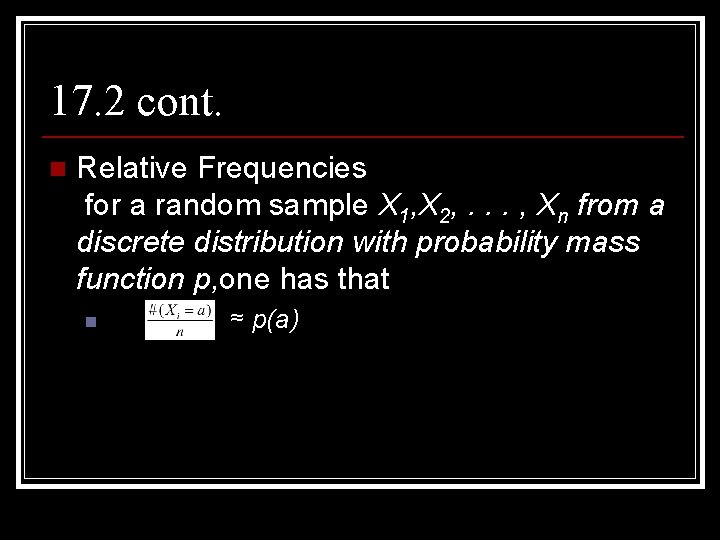17. 2 cont. n Relative Frequencies for a random sample X 1, X 2, . . . , Xn from a discrete distribution with probability mass function p, one has that n ≈ p(a)17. 4 The linear regression model n Simple Linear Regression Model: In a simple linear regression model for a bivariate dataset (x 1, y 1), (x 2, y 2), …, (xn, yn), we assume that x 1, x 2, …, xn are nonrandom and that y 1, y 2, …, yn are realizations of random variables Y 1, Y 2, …, Yn satisfying n n Yi = α + βxi + Ui for i = 1, 2, …, n, Where U 1, …, Un are independent random variables with E[Ui] = 0 and Var(Ui) = σ2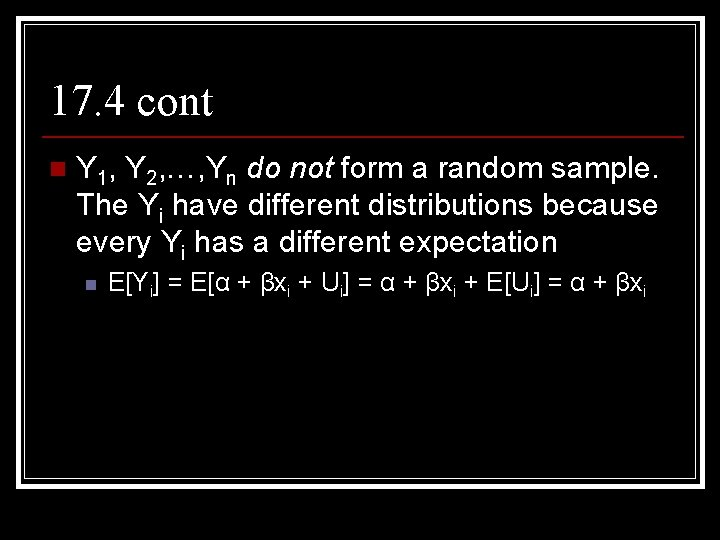17. 4 cont n Y 1, Y 2, …, Yn do not form a random sample. The Yi have different distributions because every Yi has a different expectation n E[Yi] = E[α + βxi + Ui] = α + βxi + E[Ui] = α + βxi